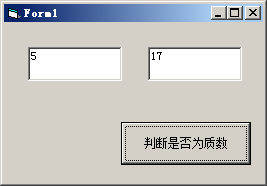# VB编程：试编程判断给定的两个正整数是否互质 vb6.0 源码

Private Sub Command1_Click()
Dim intA As Integer
Dim intB As Integer

intA = Int(Text1.Text)
intB = Int(Text2.Text)

For i = 2 To intA - 1
If (intA Mod i) = 0 Then
If (intB Mod i) = 0 Then
MsgBox "输入的两个数不是互质数", , "提示"
Exit Sub
End If
End If
Next i

For i = 2 To intB - 1
If (intB Mod i) = 0 Then
If (intA Mod i) = 0 Then
MsgBox "输入的两个数不是互质数", , "提示"
Exit Sub
End If
End If
Next i
MsgBox "输入的两个数是互为质数", , "提示"
End Subvisual basic 6.0 编程代码

<< 上一篇 下一篇 >>